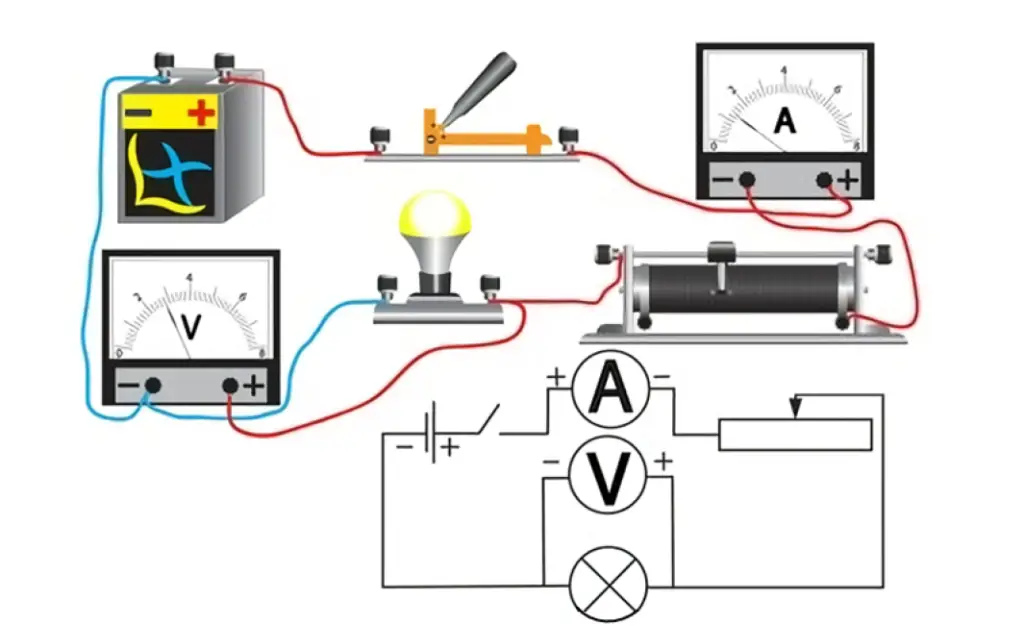# Connecting ammeter and voltmeter in physics lab

In this post, we will study a circuit diagram with ammeter and voltmeter. The goal would be to understand how to connect these meters in the circuit given in the Physics lab. You may go through our article on Ammeter and Voltmeter definition and connection if you want to revise the basics.

Let’s study the following diagram.Figure 1: (a) Ammeter and voltmeter connection in a circuit. (b) Circuit diagram with ammeter and voltmeter.

Voltmeter Connection

Figure 1 shows a voltmeter connected in parallel with a resistor (Lamp). (2 leads of Voltmeter are connected across the lamp)
Also, One lead of the voltmeter is connected to one end of the battery and the other lead is connected to the opposite end of the battery via the rest of the circuit.

A parallel connection is used because objects in parallel experience the same potential difference. Since the resistance of a voltmeter is high, it draws minimum current from the main circuit and, thus, the potential difference of the component that is going to be measured is not affected.
If a voltmeter is connected in series, it would increase the equivalence resistance of the circuit and no current would flow through the circuit. Hence, it should be connected in parallel.

Ammeter Connection

To measure the current of a circuit, the ammeter is connected in series in the circuit as shown in Figure 1 so that the same current that is there in the circuit flows through it and gets measured. The ammeter has low (nearly zero) resistance because you do not want to change the current that is flowing through the circuit. So its inclusion in series in the circuit does not change the resistance and hence the main current in the circuit. A series connection is used because objects in series have the same current passing through them. All of the current in this circuit flows through the ammeter.

See also  Series Circuits, Resistors in series & equivalent resistance formula & numerical

If an ammeter is connected in parallel, it would draw most of the current and would get damaged. Hence, it must be connected in series.

Summary

Scroll to top
error: physicsTeacher.in